# How to count and sum cells by color in Excel

I this article you will learn how to count cells by color in Excel and get the sum of colored cells. These solutions work both for cells colored manually and with conditional formatting. You will also learn how to filter cells by several colors in Excel 2010, Excel 2013, Excel 2016, and Excel 2019.

If you actively use diverse fill and font colors in your Excel worksheets to differentiate between various types of cells or values, you may want to know how many cells are highlighted in a certain color. If your cells' values are numbers, you may also want to automatically calculate the sum of cells shaded with the same color, e.g. the sum of all red cells.

As all of us know, Microsoft Excel provides a variety of formulas for different purposes, and it would be logical to assume that there are some to count cells by color. But regrettably, there is no formula that would let us sum by color or count by color in a usual Excel worksheet.

Apart from using third-party add-ins, there is only one possible solution - utilize User Defined Functions. If you know very little about this technology or have never heard this term before, don't be afraid, you will not have to write the code yourself. You will find the perfect code (written by our Excel guru) here and all that you will have to do is copy / paste it into your workbook.

## How to count by color and sum by color in an Excel worksheet

Suppose you have a table listing your company's orders where the cells in the Delivery column are colored based on their value - "Due in X Days" cells are orange, "Delivered" items are green and "Past Due" orders are red.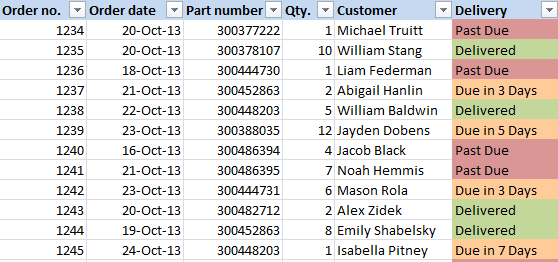What we want now is automatically count cells by color, i.e. calculate the number of red, green and orange cells in the worksheet. As I explained above, there is no straightforward solution to this task. But luckily we have very skilled and knowledgeable Excel gurus in our team and one of them has written the faultless code for Excel 2010, 2013 and 2016. So, move on with the 5 quick steps below and you will know the number and sum of your color cells in a few minutes.

1. Open your Excel workbook and press Alt+F11 to open Visual Basic Editor (VBE).
2. Right-click on your workbook name under "Project-VBAProject" in the right hand part of the screen, and then choose Insert > Module from the context menu.```Function GetCellColor(xlRange As Range)
Dim indRow, indColumn As Long
Dim arResults()

Application.Volatile

If xlRange Is Nothing Then
Set xlRange = Application.ThisCell
End If

If xlRange.Count > 1 Then
ReDim arResults(1 To xlRange.Rows.Count, 1 To xlRange.Columns.Count)
For indRow = 1 To xlRange.Rows.Count
For indColumn = 1 To xlRange.Columns.Count
arResults(indRow, indColumn) = xlRange(indRow, indColumn).Interior.Color
Next
Next
GetCellColor = arResults
Else
GetCellColor = xlRange.Interior.Color
End If
End Function

Function GetCellFontColor(xlRange As Range)
Dim indRow, indColumn As Long
Dim arResults()

Application.Volatile

If xlRange Is Nothing Then
Set xlRange = Application.ThisCell
End If

If xlRange.Count > 1 Then
ReDim arResults(1 To xlRange.Rows.Count, 1 To xlRange.Columns.Count)
For indRow = 1 To xlRange.Rows.Count
For indColumn = 1 To xlRange.Columns.Count
arResults(indRow, indColumn) = xlRange(indRow, indColumn).Font.Color
Next
Next
GetCellFontColor = arResults
Else
GetCellFontColor = xlRange.Font.Color
End If

End Function

Function CountCellsByColor(rData As Range, cellRefColor As Range) As Long
Dim indRefColor As Long
Dim cellCurrent As Range
Dim cntRes As Long

Application.Volatile
cntRes = 0
indRefColor = cellRefColor.Cells(1, 1).Interior.Color
For Each cellCurrent In rData
If indRefColor = cellCurrent.Interior.Color Then
cntRes = cntRes + 1
End If
Next cellCurrent

CountCellsByColor = cntRes
End Function

Function SumCellsByColor(rData As Range, cellRefColor As Range)
Dim indRefColor As Long
Dim cellCurrent As Range
Dim sumRes

Application.Volatile
sumRes = 0
indRefColor = cellRefColor.Cells(1, 1).Interior.Color
For Each cellCurrent In rData
If indRefColor = cellCurrent.Interior.Color Then
sumRes = WorksheetFunction.Sum(cellCurrent, sumRes)
End If
Next cellCurrent

SumCellsByColor = sumRes
End Function

Function CountCellsByFontColor(rData As Range, cellRefColor As Range) As Long
Dim indRefColor As Long
Dim cellCurrent As Range
Dim cntRes As Long

Application.Volatile
cntRes = 0
indRefColor = cellRefColor.Cells(1, 1).Font.Color
For Each cellCurrent In rData
If indRefColor = cellCurrent.Font.Color Then
cntRes = cntRes + 1
End If
Next cellCurrent

CountCellsByFontColor = cntRes
End Function

Function SumCellsByFontColor(rData As Range, cellRefColor As Range)
Dim indRefColor As Long
Dim cellCurrent As Range
Dim sumRes

Application.Volatile
sumRes = 0
indRefColor = cellRefColor.Cells(1, 1).Font.Color
For Each cellCurrent In rData
If indRefColor = cellCurrent.Font.Color Then
sumRes = WorksheetFunction.Sum(cellCurrent, sumRes)
End If
Next cellCurrent

SumCellsByFontColor = sumRes
End Function```
4. Save your workbook as "Excel Macro-Enabled Workbook (.xlsm)".

If you are not very comfortable with VBA, you can find the detailed step-by-step instructions and a handful of useful tips in this tutorial: How to insert and run VBA code in Excel.

5. Now that all "behind the scenes" work is done for you by the just added user-defined function, choose the cell where you want to output the results and enter the CountCellsByColor function into it:

CountCellsByColor(range, color code)

In this example, we use the formula `=CountCellsByColor(F2:F14,A17)` where F2:F14 is the range containing color-coded cells you want to count and A17 is the cell with a certain background color, a red one in our case.

In a similar way, you write the formula for the other colors you want to count, yellow and green in our table.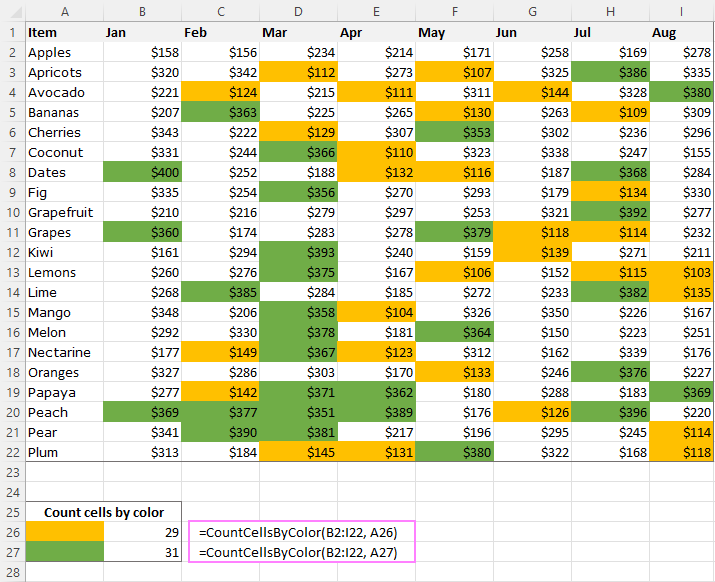If you have numerical data in colored cells (e.g. the Qty. column in our table), you can add up the values based on a certain color by using an analogous SumCellsByColor function:

SumCellsByColor(range, color code)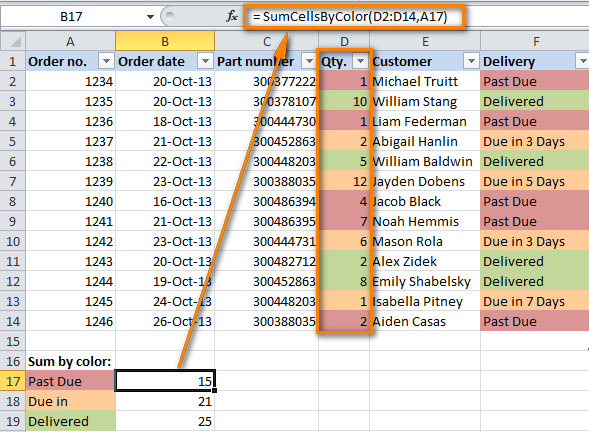As demonstrated in the screenshot above, we used the formula `=SumCellsByColor(D2:D14,A17)` where D2:D14 is the range and A17 is the cell with a color pattern.

In a similar way you can count cells and sum cells' values by font color using the `CountCellsByFontColor` and `SumCellsByFontColor` functions, respectively.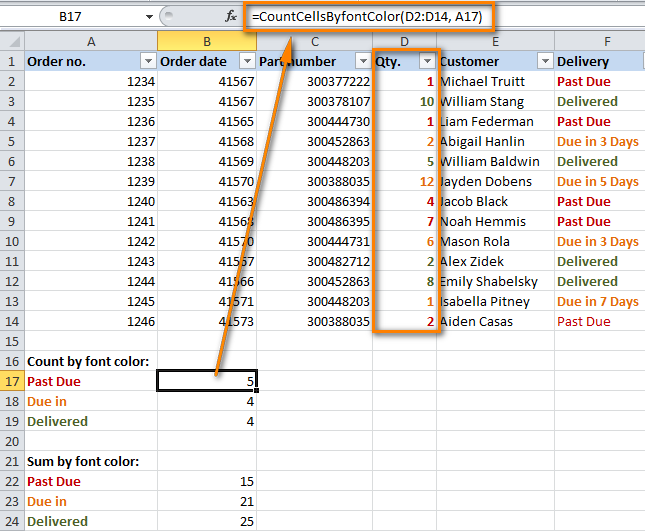Note: If after applying the above mentioned VBA code you would need to color a few more cells manually, the sum and count of the colored cells won't get recalculated automatically to reflect the changes. Please don't be angry with us, this is not a bug of the code : )
In fact, it is the normal behavior of all Excel macros, VBA scripts and User-Defined Functions. The point is that all such functions are called with a change of a worksheet's data only and Excel does not perceive changing the font color or cell color as a data change. So, after coloring cells manually, simply place the cursor to any cell and press F2 and Enter, the sum and count will get updated. The same applies to the other macros you will find further in this article.

## Sum by color and count by color across the entire workbook

The VB script below was written in response to Connor's comment (also by our Excel's guru Alex) and does exactly what Connor requested, namely counts and sums the cells of a certain color in all worksheets of the workbook. So, here comes the code:

```Function WbkCountCellsByColor(cellRefColor As Range)
Dim vWbkRes
Dim wshCurrent As Worksheet

Application.ScreenUpdating = False
Application.Calculation = xlCalculationManual

vWbkRes = 0
For Each wshCurrent In Worksheets
wshCurrent.Activate
vWbkRes = vWbkRes + CountCellsByColor(wshCurrent.UsedRange, cellRefColor)
Next
Application.ScreenUpdating = True
Application.Calculation = xlCalculationAutomatic

WbkCountCellsByColor = vWbkRes
End Function

Function WbkSumCellsByColor(cellRefColor As Range)
Dim vWbkRes
Dim wshCurrent As Worksheet

Application.ScreenUpdating = False
Application.Calculation = xlCalculationManual

vWbkRes = 0
For Each wshCurrent In Worksheets
wshCurrent.Activate
vWbkRes = vWbkRes + SumCellsByColor(wshCurrent.UsedRange, cellRefColor)
Next
Application.ScreenUpdating = True
Application.Calculation = xlCalculationAutomatic

WbkSumCellsByColor = vWbkRes
End Function```

You use this macro in the same manner as the previous code and output the count and sum of the colored cells with the help of the following formulas, `=WbkCountCellsByColor()` and `=WbkSumCellsByColor()`, respectively. Simply enter either formula in any empty cell on any sheet without defining a range, specify the address of any cell of the needed color in brackets, e.g. =WbkSumCellsByColor(A1), and the formula will display the sum of all the cells shaded with the same color in your workbook.

### Custom functions to get a cell's background color, font color and color code

Here you will find a summary of all the functions we've used in this example as well as a couple of new ones that retrieve color codes.

Note: Please remember that all of these formulas will work only if you have added the user-defined function to your Excel workbook as demonstrated earlier in the article.
##### Functions to count by color:
• `CountCellsByColor(range, color code)`- counts cells with the specified background color.

In the above example, we used the following formula to count cells by color =CountCellsByColor(F2:F14,A17) where F2:F14 is the selected range and A17 is the cell with the needed background color. You can use all other formulas listed below in a similar way.

• `CountCellsByFontColor(range, color code)` - counts cells with the specified font color.
##### Formulas to sum by color:
• `SumCellsByColor(range, color code)` - calculates the sum of cells with a certain background color.
• `SumCellsByFontColor(range, color code)` - calculates the sum of cells with a certain font color.
##### Formulas to get the color code:
• `GetCellFontColor(cell)` - returns the color code of the font color of a specified cell.
• `GetCellColor(cell)` - returns the color code of the background color of a specified cell.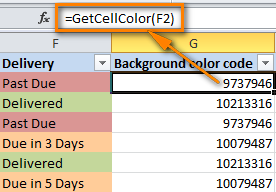Well, counting cells based on color and getting the sum of colored cells was pretty easy, wasn't it? Of course if you have that little VBA gem that makes the magic happen : ) But what if you do not color cells manually and rather use conditional formatting, as we discussed in these two articles How to change the background color of cells and How to change a row's color based on cell value?

## How to count by color and sum cells colored using conditional formatting

If you have applied conditional formatting to color cells based on their values and now you want to count cells by color or sum the values in colored cells, I have bad news - there is no universal user-defined function that would sum by color or count color cells and output the resulting numbers directly in the specified cells. At least, I am not aware of any such function, alas : (

Of course, you can find tons of VBA code on the Internet that attempts to do this, but all those codes (at least the examples I've come across, do not process conditional formatting such as "Format all cells based on their values", "Format only top or bottom ranked values", "Format only values that are above or below average", "Format only unique or duplicate values". Besides that nearly all those VBA codes have a number of specificities and limitations because of which they may not work correctly with certain workbooks or data types. All in all, you can try your luck and google for an ideal solution and if you happen to find one, please do come back and post your finding here!

The VBA code below overcomes the above mentioned limitations and works in Microsoft Excel 2010, Excel 2013 and Excel 2016 spreadsheets with all types of condition formatting (kudos to Alex again!). As a result, it displays the number of colored cells and the sum of values in those cells, no matter which type of conditional formats are used in a sheet.

```Sub SumCountByConditionalFormat()
Dim indRefColor As Long
Dim cellCurrent As Range
Dim cntRes As Long
Dim sumRes
Dim cntCells As Long
Dim indCurCell As Long

cntRes = 0
sumRes = 0

cntCells = Selection.CountLarge
indRefColor = ActiveCell.DisplayFormat.Interior.Color

For indCurCell = 1 To (cntCells - 1)
If indRefColor = Selection(indCurCell).DisplayFormat.Interior.Color Then
cntRes = cntRes + 1
sumRes = WorksheetFunction.Sum(Selection(indCurCell), sumRes)
End If
Next
MsgBox "Count=" & cntRes & vbCrLf & "Sum= " & sumRes & vbCrLf & vbCrLf & _
"Color=" & Left("000000", 6 - Len(Hex(indRefColor))) & _
Hex(indRefColor) & vbCrLf, , "Count & Sum by Conditional Format color"
End Sub```

### How to use the code to count colored cells and sum their values

1. Add the above code to your worksheet as explained in the first example.
2. Select a range or ranges where you want to count colored cells or/and sum by color if you have numerical data.
3. Press and hold Ctrl, select one cell with the needed color, and then release the Ctrl key.
4. Press Alt+F8 to open the list of macros in your workbook.
5. Select the SumCountByConditionalFormat macro and click Run.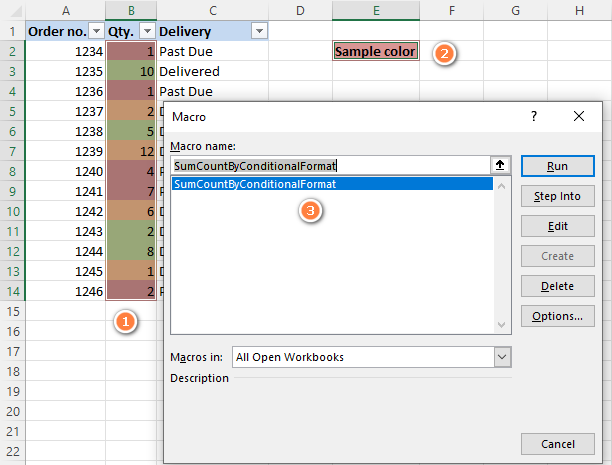As a result, you will see the following message: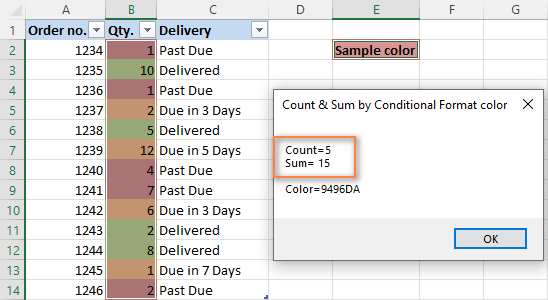For this example, we selected the Qty. column and got the following numbers:

• Count is the number of the cells with a particular color, a reddish color in our case that marks "Past Due" cells.
• Sum is the sum of values of all red cells in the Qty. column, i.e. the total number of "Past Due" items.
• Color is the Hexadecimal color code of a selected cell, D2 in our case.

If you have any difficulties with adding the scripts to your Excel workbooks, such as compilation errors, formulas not working and so on, please download this sample workbook with the CountCellsByColor and SumCellsByColor functions ready for use and try them on your data.

## Fastest way to count and sum cells by color in Excel

Updated on 1-Jul-2014

When we published this article, we hoped it would be popular because we used to get a lot of questions about how to count and sum cells by color in Excel. But even in our wildest expectations, we did not think it was going to be that popular! What you see in comments on this page is just a tiny portion of the enormous feedback we have received. So, our team decided to take a step further and create an Excel add-in that would count and sum cells by the color you specify or by all colors in the selected range.

Let me introduce you to our brand new tool - Count & Sum by Color for Excel 2019 - 2007. Once installed, it will place 2 buttons onto the Ablebits Data tab on your Excel ribbon - One Color and All Colors, as you can see in the screenshot below: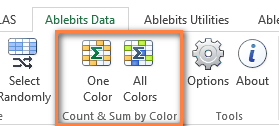### Count and sum cells by the selected color

You simply click the One Color button on the ribbon and have the Count & Sum by Color pane open at the left of the worksheet. On the pane, you select:

• The range where you want to count and sum the cells
• Any color-coded cell
• Color option - either background or font color

Once done, click Calculate and see the result in the lower part of the pane straight away! Apart from the count and sum, the add-in calculates the average and finds the max and min values. No macros, no formulas, no pain :)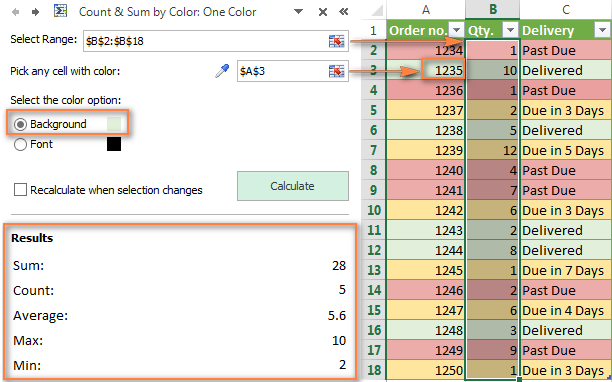### Count and sum cells by all colors in the selected range

The All Colors option works basically in the same way, except that you do not have to choose the color. In the "Show results for" section, you can select any of the following options: Count, Sum, Average, Maximum or Minimum value.

If you want to copy the results to your worksheet, click the Paste results... button at the bottom of the Count & Sum by Color pane.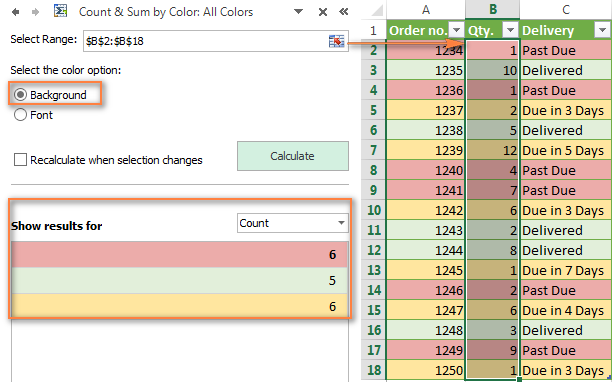And here is a list of the main features you will find in the Count & Sum by Color add-in:

• Count and sum cells by color in all versions of Excel 2016, 2013, 2010, 2007 and 2003.
• Handle cells color-coded manually and with conditional formatting.
• Besides counting and calculating the sum, the following functions are available: Average, Maximum and Mininum values.
• An option to recalculate colored cells automatically when a new range is selected.
• Copy and paste the results to any location on the current spreadsheet or to any other sheet.

### How can I get the Count & Sum by Color add-in?

Currently the add-in is available as part of the Ultimate Suite for Excel. This is a collection of our best tools especially designed to deal with the most tedious, painstaking and error-prone tasks in Excel.

In addition to the Count & Sum by Color add-in, the Ultimate Suite includes over 60 time-saving tools tools that will help you merge data from different worksheets, remove duplicates, compare sheets for matches and differences, and a lot more!

If you like the tools, don't miss this special opportunity to get a license at the lowest price:

Get Promo Code for Ultimate Suite - exclusive offer for our blog readers!

### 576 responses to "How to count and sum cells by color in Excel"

1.Nirasha says:

Thank You so much. This is really useful.

2.Sonia D Spears says:

Excel by color coding

3.Rachel Friedman says:

I tried the countcellsbycolor formula, it just plain did not work! i followed all instructions right & got the #NAME? dumb error over 7 over again.

4.Ahmed says:

i need to count multi color in big range contains of about 1000 cells could you help please

5.Ahmed says:

now it is working thanks

6.Dana says:

This was awesome and so easy to implement. Thank you so much!!!

7.James Duley says:

I have been helping my wife with some spreadsheets. Everything was going great until she needed to count employees and the new new employees were typed in red font and were not counted in the total number of employees. Your CountCellsByFontColor worked perfect.

Thank you very much!!!